﻿ Semiparametric Estimation of Receiver Operating Characteristic SurfacePublications are Open
Access in this journal
Article Versions
Export Article
• Normal Style
• MLA Style
• APA Style
• Chicago Style
Research Article
Open Access Peer-reviewed

Semiparametric Estimation of Receiver Operating Characteristic Surface

Ben K. Koech
American Journal of Applied Mathematics and Statistics. 2018, 6(6), 218-223. DOI: 10.12691/ajams-6-6-1
Received July 10, 2018; Revised August 23, 2018; Accepted October 31, 2018

Abstract

Receiver operating characteristic curve analysis is widely used in biomedical research to assess the performance of diagnostic tests. Estimation of receiver operating characteristic curves based on parametric approach has been widely used over years. However, this is limited by the fact that distribution of almost all diseases in epidemiology cannot be established quite easily. Semi parametric methods are robust as it allows computability and the distributions based on this are flexible. Furthermore, there is need for generalization of the receiver operating characteristic curve (since, the analysis largely assumes that test results are dichotomous) to allow tests to have more than two outcomes. The receiver operating characteristic curve was generalized to constitute a surface, which uses volume under the surface (VUS) to measure the accuracy of a diagnostic test. Dirichlet process mixtures of normals, which is a robust model that can handle nonstandard features in data in modelling the diagnostic data, were used to model the test outcomes. Semiparametric Dirichlet process mixtures of normals for receiver operating characteristic surface estimation were fitted using Markov Chain Monte Carlo with simple Metropolis Hastings steps. The Semi-parametric simulation results indicate that even when the parametric assumption holds, these models give accurate results as the volume under the surface (VUS) for both methods were greater than 1/6, the value of a “useless test”. Graphically, the semiparametric receiver operating characteristic surface has the appealing feature of being continuous and smooth, thus allowing for useful interpretation of the diagnostic performance at all thresholds.

1. Introduction

The difficulty in generalizing the ROC curve to more than two disease classes results from the fact that a decision rule for a K-group classification will produce K true class rates and K(K-1) false class rates. Semiparametric approach, allows these problems to be addressed directly via inference about the relevant model parameters. The model can be implemented using data distributions derived as normal mixtures in the framework of mixtures of Dirichlet processes 1. West 2 discussed these models in a special case of the framework by developing approximations to predictive distributions based on a clustering algorithm motivated by the model structure and draws obvious connections with kernel approaches. For single test designs, Erkanli et al. 3 used truncated Dirichlet process mixture models while Branscum et al. 4 developed mixture of finite Polya trees models for Bayesian nonparametric ROC data analysis when true infection status is unknown. Choi et al. 5 developed Bayesian parametric multivariate ROC methodology while Hall and Zhou 6 developed a multivariate distribution-free frequentist approach. Most applications have involved modelling univariate data because, unlike Dirichlet process priors, the initial development of PT priors focused on continuous distributions supported on the real line 7. Heckerling 8, proposed a simple parametric frequentist approach under the assumption that test results all follow normal distributions. Li and Zhou 9 considered non-parametric and semi parametric estimation of the ROC surfaces by approximating the asymptotic ROC surfaces with multivariate Brownian bridge processes. The semiparametric approach attempts to generalize a parametric (normal) functional form of the ROC surface. However, this latter approach, as pointed out by the authors, relies heavily on the normality assumption. Parametric models are often not sufficiently flexible to capture skewness, multimodality, or other nonstandard features of the data. However, this generalization has the potential to make the inference robust to departures from an assumed parametric distribution while still having good performance if the actual distribution is the parametric one.

This study sought to complement the modelling of diagnostic data of the test measurement using a three-sample density ratio model. The advantage of applying Dirichlet process mixtures into the ROC surface analysis is that it not only allows estimation of the ROC surface semi parametrically but also enables implementation of the method easy as the usual procedures in many statistical software packages can be employed.

2. Methods

Suppose that the normal means and variances,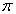come from some prior distribution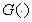on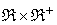. Ifis uncertain and modeled as a Dirichlet process, then the data come from a Dirichlet mixture of normals 4, 6, 10.

In particular, it is supposed that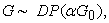a Dirichlet process defined by, a positive scalar, and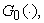a specified bivariate distribution function over.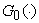is the prior expectation of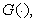so thatfor allandis a precision parameter, determining the concentration of the prior forabout.Write parameters of concern as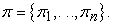A key feature of the model structure, and of its analysis, relates to the discreteness ofunder the Dirichlet process assumption 11 Briefly, in any sampleof size n fromthere is positive probability of coincident values. Thus, given, a sample of size n from, the subsequent estimates represents a new, distinct probability values. In practical density estimation, suitable values ofwill typically be small relative to the initial prior. Dirichlet process mixture models are based on Dirichlet process priors for the primary parameters.

Such a model assumes that the prior distribution function itself is uncertain drawn from a Dirichlet process in standard notation such as in Antoniak 10. Hsieh and Turnbull 12 considered similar estimation methodology for binary ROC curves. For this research, the methodology was extended to accommodate three group test outcomes as a generalization of the binary ROC curves.

A generic function that performs ROC surface analysis based on Dirichlet process mixture of normals models for density estimation was considered 13. A diagnostic test in which subjects can be classified into three different ordered categories was assumed.

Considering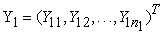test results on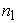subjects from Class 1;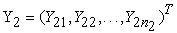test results onsubjects from Class 2; and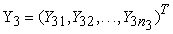test results onsubjects from Class 3.

The test results for the individuals from the three classes are modeled according to the distribution,and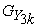; continuous probability distributions on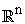. Functional form of Dirichlet Process Mixtures of Normals for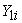is given by: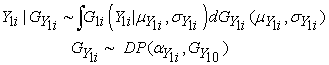This can be substituted for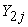andas well. The distribution for(distribution for the non-diseased or healthy group) can be expressed as follows: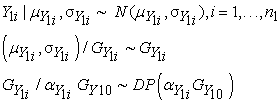(1)

where, the baseline distribution is the conjugate normal-inverted-Wishart,Similarly for(distribution for the transition or suspicious group)(2)

It was assumed the baseline distribution is the conjugate normal-inverted-Wishart,Finally, for(distribution for the diseased group)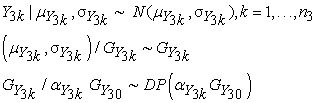(3)

It was also assumed that the baseline distribution is the conjugate normal-inverted-Wishart,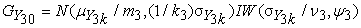To let part of the baseline distribution fixed at a particular value, the corresponding hyperparameters of the prior distributions were set to null in the hyperprior specification of the model.

Although the baseline distribution,,and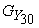, are conjugate priors in the model specifications, the algorithms with auxiliary parameters described in MacEachern and Muller 14 and Neal 15 are adopted.

The ROC surface using a Monte Carlo approximation to the posterior means,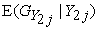, and, which is based on MCMC samples from posterior predictive distribution for a future observation.,andare the vectors containing the diagnostic marker measurements in the non-diseased, transition or suspicious group and diseased subjects, respectively. The optimal cut-off point is based on the efficiency test, EFF = TP + TTR+TN, and is built on Cohen’s kappa as defined in Kraemer 16.

3. Analysis of Data and Results

The simulation study to assess the performance of the Non-parametric estimation method covers test scores for the three classes generated from three different normal populations: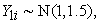and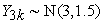, where sample sizesand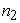, and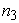were set to 100, 50 and 100. The means are ordered to ensure that the observations are monotonically increasing while the sample sizes were considered under conditions for diagnostic tests in clinical practice 17. To fit the Semiparametric density estimation, Dirichlet Process Mixtures (DPM) of normals model for density estimation was used. The prior parameters are defined as;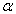=1 gives the value of the precision parameter,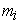replicated for the values i=1,2 and 3 gives the mean of the normal part of the baseline distribution. In addition,of a solution of scale matrix gives the hyperparameters of the inverted Wishart prior distribution for the scale matrix,, of the inverted Wishart part of the baseline distribution. Further,represents the hyperparameters of the inverted Wishart part of the baseline distribution.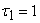and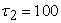gives the hyperparameters for the gamma prior distribution of the scale parameter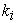of the normal part of the baseline distribution. Theand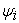parameters replicated for the values 1, 2 and 3 fitted in the posterior distribution represents the scale parameter of the normal part of the baseline distribution and the scale matrix of the inverted-Wishart part of the baseline distribution respectively.

3.1. Data Plots under Semiparametric Estimation

Plots of posterior parameters;and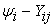showed that the processes appear to be stationary. All plots suggested that convergence was achieved after 1000 iterations of the Metropolis Hastings steps Sampler. Inference thereof is that each plot seemed to confirm that the parameter posterior parameters converged to stationarity after 1000 or so iterations. Further, the fitted distribution was analysed by comparing it with the data plots and kernel density estimate plots. It was shown that the posterior distribution curve- DPM of normals model curve fit and kernel density estimate curve fit for the data. The kernel density estimate curve fits for the posterior estimates represented by faint line while dark line represented the posterior distribution curve fit.

Distribution of(test outcomes for the nondiseased group) under Semiparametric estimation

The data plot for(test outcomes for the non-diseased group) was represented using a histogram. The posterior distribution of the test outcomes for the non-diseased group and curve fits for DPM of normals model and kernel density estimate were also fitted on the same data plot. Figure 1 below shows the data plot, DPM of normals model curve fit and kernel density estimate curve fit.

• Figure 1. Distribution of (test outcomes for the nondiseased group) under Semiparametric estimation

Figure 1 above shows that plot for Y1i (test outcomes for the nondiseased group) portrays that the data assumes some distribution, evident in the presence of peaks. The DPM of normals model posterior distribution fit for the test outcomes is a near symmetric curve fit indicating that the posterior distribution fits the data well. The kernel density smooth curve fit further confirmed that that the DPM of normals model fits the data convincingly.

Distribution of(test outcomes for the transition or suspicious group) under Semiparametric estimation

The plots for(test outcomes for the transition or suspicious group) were computed using a histogram. The posterior distribution of the test outcomes for the transition or suspicious group and curve fits for DPM of normals model and kernel density estimate were also fitted on the same data plot. DPM of normals model curve fit and kernel density estimate curve fit are shown in Figure 2 below.

• Figure 2. Distribution of (test outcomes for the transition or suspicious group) under Semiparametric estimation

The presence of peaks in data plot for(test outcomes for the transition or suspicious group) portrays that the test outcomes assumes some distribution. The DPM of normals model posterior distribution fit for these test outcomes was a near perfect symmetric curve or normal distribution fit indicating that the posterior distribution fits the data well. The kernel density smooth curve fit further confirmed that that the DPM of normals model fits the data convincingly, especially a true parameter values.

Distribution of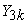(test outcomes for the diseased group) under semiparametric estimation

The data for(test outcomes for the diseased group) was also plotted using a histogram. The posterior distribution of the test outcomes for the diseased group and curve fits for DPM of normals model and kernel density estimate were also fitted on the same data plot. DPM of normals model curve fit and kernel density estimate curve fit are shown in Figure 3 below.

• Figure 3. Distribution of (test outcomes for the diseased group) under Semiparametric estimation

The histogram or data plot representing posterior distribution of(test outcomes for the diseased group) reveals that the test outcomes follow some distribution. The fit The DPM of normals model posterior distribution for these test outcomes lacked smooth fit though it exhibited peak at true parameter values. The kernel density smooth curve fit and the DPM of normals model curve fit were found to be adjacent indicating that the DPM of normals model fits the data well.

3.2. Semiparametric Estimation of ROC Surface

Having seen that the properties semiparametric density estimators; the posterior parameters were desirable, samples were drawn to estimate the ROC surface. Random samples for the three test outcomes using the DPM of Normals procedures. These data represents test outcomes of a simulated diagnostic test that classifies disease or condition into three ordered groups namely D- (non diseased), D0 (transition or suspicious) and D+ (diseased). It was assumed that the test outcomes are ordinal and that the simulated diagnostic test classifies the groups without overlap. Table 1 below provides a summary of the test outcomes drawn from the distribution.

ROC surface plot

From the simulated diagnostic test classification of test outcomes, a three way ROC surface corresponding to the three test outcomes was plotted. The Semiparametric model defined in to estimate the ROC surface plot were used. Figure 9 below represents three dimensional surface plot depicting trade-offs between the predictive measures for classification of the three test outcomes.

• Figure 4. Three-dimensional ROC surface plot depicting tradeoffs between the predictive measures for classification of the three test outcomes under semiparametric model

Figure above represents a plot to estimate the ROC surface under the semiparametric model assumptions. It was evident that the semiparametric model gives a near smooth ROC surface indicating that the ROC surface has good coverage thus the simulated diagnostic test performs well in classifying the test outcomes.

3.3. Semiparametric Estimation of Volume under ROC Surface (VUS)

For the simulated diagnostic test that classified the test outcomes into the three groups to estimate the volume under ROC surface, volume under surface group of test outcomes under the Semiparametric assumptions based on DPM of normals model was computed. It was found out that the volume under the surface VUS= 0.3411. The 95% confidence interval was also computed where the lower confidence interval was found to be 0.317 while the upper confidence interval was found to be 0.4598.

Additionally, optimal cut-points off points were computed whereby the best lower cut-point was found to be 0.0935 while the upper cut-point was found to be 0.2307. The group correct classification probabilities were found to be; specificity= 0.48, true transition rate=0.74 and sensitivity=0.42. It was also derived that the estimate of the sample size=115 for the predefined precision. As such, to better estimate the diagnostic accuracy of the marker or group of test outcomes, minimum sample size of 115 will be desired for each group in order to estimate the VUS of the marker within a 5% margin of error.

3.4. Scatter Plot and a Boxplot for the Semi-Parametric VUS

To provide a summary of the semiparametric volume under ROC surface, a general scatter plot and a boxplot were plotted. The graphical summary of the data for D- , D0 and D+ colored in green, blue and red, respectively and the estimated summary measure for the confidence interval (CI) is provided in the legend while the optimal cut-points are labeled for the Semiparametric VUS. Figure 10 below gives the summary.

• Figure 5. Scatter plot and a boxplot of the marker under semiparametric model

From Figure 5 above, the dashed lines show the lower and uppercut points for the Semiparametric VUS. The value of the VUS 0.34 is also shown. The box plots portrays that the test outcomes show some ordering and that the test outcomes are ordinal or values are monotonically increasing.

4. Discussion

The simulation studies were conducted to assess the semiparametric estimator of ROC surface. In every simulation, true-negative, true transition or suspicious and true-positive samples for the two methods were generated. Samples for the semiparametric approach; the true-negative class, true transition or suspicious and the true-positive class follows normal distributions with standard deviation of 1.5 with varying mean values. The set of simulations, test outcomes were assumed to have different sample sizes according to definite class ratio 2:1:2 as recommended by 18. As expected, the methods perform better when the sample size is larger. The estimators had some drawbacks, and it may suffer from large variability, particularly for small sample sizes. However, this is not a major problem as small samples are common-place in clinical practice 17.

The parameter plots suggested that the Metropolis Hastings steps sampler used achieved convergence and were valid for subsequent analysis. It was deduced from the trace plots for all parameters that inference based on the parameters was robust. In particular, the semiparametric posterior parameters were all stationary at the true parameter values and that all the MCMC chains were all convergent. That is, the high acceptance rate does indicate that the algorithm is behaving satisfactorily since it correspond to the fact that the chain is moving too faster on the surface. The plots for the posterior distributions for the three test outcomes under the Semiparametric case (DPM of normals) were all smooth and symmetric except forwhich had asymmetric data plot; this can be attributed to the small sample size used. It was noted that the Kernel Density Estimation for both Semiparametric and non-parametric posterior distributions tend toward the true density therefore it is convincing that a much narrower variation on the sample values can be obtained. The Semiparametric posterior distribution fit was found to have smooth and more adjacent fit to kernel density estimate fits compared to the nonparametric posterior distribution fits.

As such, the Semiparametric estimator provides a good mathematical model under assumed population distributions for the test results in three classes. It was also noticed that the choice of normal distribution in semiparametric model may be replaced by other well-known parametric families such as Weibull or gamma, where appropriate. The results compared with the semiparametric approach of Carvalho et al. 19 shows that the model performs competitively. The Bayesian nonparametric approach developed as based on mixtures of finite Polya trees priors outperforms the estimators proposed by Inácio 20. However, the developed methods are prone to overlap between test outcomes. The ROC surface under the Semiparametric and nonparametric models were found to have good coverage thus the two surfaces was considered useful to examine the diagnostic accuracy of the test for the three classes at different threshold values. The computed semiparametric ROC surface had similar methods used by Nakas and Yiannoutsos 21. The estimated VUS under Semiparametric model was 0.34 and a 95% credible interval was (0.317, 0.460). On the other hand, the estimated VUS under Nonparametric model was 0.26 and a 95% credible interval was (0.232, 0.204). These values was contrasted to the VUS of a useless test or uninformative level of VUS=1/6 =0.167, which led us to conclude that the assumed marker has a reasonable discriminative power under both model assumptions. From these results, it can be concluded that for this particular analysis all methods lead substantially to the same conclusions. Even with small sample sizes, the DPM of normals model behaves quite well. Moreover, it was experienced that these approaches fits normal data well and is robust enough to fit data generated from other distributions for example gamma and for exponential distributions as mixtures of such distributions.

It was also noted that full inference is available with the Bayesian approach employed since the pair of thresholds that should be used to make the diagnostic decision was obtained. Nakas et al. 22 earlier suggested that once models have been fitted, a posterior distribution for the optimal thresholds can easily be obtained.

5. Conclusion

In summary, diagnostic tests for three ordinal groups are important in biomedical practice. A useful summary measure (VUS) which can be adopted to evaluate the discriminative ability of a diagnostic test when there are three ordinal groups was computed. The applicability of DPM model in solving difficulty in the modelling of continuous diagnostic data with skewness, multimodality or other nonstandard features were discussed. These data-driven models provided robust inference for the ROC surface and for the volume under the ROC surface (VUS). Further, the summary of ROC surface under the semiparametric case indicated that the method perform equally well in the simulation studies. The produced ROC surface plots has the appealing feature of being continuous and smooth, thus allowing for useful interpretation of the diagnostic performance at all thresholds. The surface plots produced are useful to examine the diagnostic accuracy of the test for the three classes at different threshold values. Notably, the volume under surface for semiparametric model depicted relatively higher accuracy which can be attributed to the fact that the semiparametric model is largely centred on the Gaussian distribution. It is noteworthy that semiparametric modelling of diagnostic data does not mean that there are no parameters in the model that was assumed was massively parametric as the posterior parameters for the models were fitted. The terms were used to indicate that the models are free of restrictive, inappropriate constraints that are implied particular parametric models. This study recommends for a generalization of more than three classes (K > 3), to produce a ROC hypersurface. The Hypervolume Under the ROC Manifold (HUM) can be used for inferences for the ROC manifold especially in genetics where gene classifications involve several categories. It is also recommends that researchers who are interested ROC surface methodology use the Comprehensive R Archive Network repository and contribute to further developments of R-packages for the implementation of ROC surface analysis in order to facilitate more researchers to implement ROC surface methodology.

References

  Ferguson, T. S (1983). Bayesian Density Estimation by Mixtures of Normal Distributions, in Recent Advances in Statistics, eds. H. Rizvi and J. Rustagi, New York: Academic Press. In article  West, M. (1990). Bayesian kernel density estimation. Institute of Statistics and Decision Sciences, Duke University. In article  Erkanli, A., Sung, M., Costello, E.J., & Angold, A. (2006). Bayesian semi-parametric ROC analysis. Statistics in Medicine. 25, 3905-3928. In article View Article  PubMed  Branscum, A. J., Johnson, W. O., Hanson, T. E. & Gardner, I. A. (2008). Bayesian semiparametric ROC curve estimation and disease diagnosis. Statistics in Medicine. 27, 2474-2496. In article View Article  PubMed  Choi, Y., Johnson, W.O., Gardner, I. A., & Collins, M.T. (2006). Bayesian inference for receiver operating characteristic curves in the absence of a gold standard. Journal of Agricultural Biology and Environmental Statistics. 11: 210-229. In article View Article  Hall, P. & Zhou, X. H. (2003). Nonparametric estimation of component distributions in a multivariate mixture. Annals of Statistics.31, 201-224. In article View Article  Ferguson, T. S. (1974). Prior distributions on spaces of probability measures. The Annals of Statistics 2, 615-629. In article View Article  Heckerling, P. S. (2001). Parametric three-way receiver operating characteristic surface analysis using Math-ematica. Medical Decision Making. 20, 409-417. In article View Article  Li, J., Zhou, X.H. (2009). Nonparametric and Semiparametric Estimation of the Three Way Receiver Operating Characteristic Surface. Journal of Statistical Planning and Inference. 139, 4133-4142. In article View Article  Antoniak, C. E. (1974). Mixtures of Dirichlet processes with applications to Bayesian nonparametric problems. Annals of Statistics, 2, 1152-74. In article View Article  Ferguson, T.S.(1973). A Bayesian Analysis of Some Nonparametric Problems. The Annals of Statistics, (1), 209-230. In article View Article  Hsieh, F. & Turnbull, B.W. (1996). Nonparametric and semiparametric estimation of the receiver operating characteristic curve. Annals of Statistics. (24), 25-40. In article View Article  Escobar, M.D. & West, M. (1995) Bayesian Density Estimation and Inference Using Mixtures. Journal of the American Statistical Association, 90: 577-588. In article View Article  MacEachern, S. N. & Muller, P. (1998). Estimating mixture of Dirichlet Process Models. Journal of Computational and Graphical Statistics, 7 (2): 223-338. In article  Neal, R. M. (2000). Markov Chain sampling methods for Dirichlet process mixture models. Journal of Computational and Graphical Statistics, 9: 249-265. In article  Kraemer, H. C. (1992). Evaluating Medical Tests. Sage Publications. In article  Jokiel-Rokita, A. & Pulit, M. (2013). Nonparametric estimation of the ROC curve based on smoothed empirical distribution functions, Statistics andComputing, 23, 703-712. In article  Luo, J. & Xiong, C. (2012). DiagTest3Grp: An R Package for Analyzing Diagnostic Tests with Three Ordinal Groups. Journal of Statistical Software. 51(3), 1-24. In article View Article  PubMed  Inacio de Carvalho, V., Jara, A., Hanson, T. E. & de Carvalho, M. (2013). Bayesian nonparametric ROC regression modeling, Bayesian Analysis, 3, 623-646. In article View Article  Inácio V, Turkman AA, Nakas CT, Alonzo TA. (2012) Nonparametric Bayesian Estimation of the Three-Way Receiver Operating Characteristic Surface. Biometrical Journal. 53(6): 1011-1024. In article View Article  PubMed  Nakas, C. T. and Yiannoutsos, C. T. (2004). Ordered multiple-class ROC analysis with continuous measurements. Statistics in Medicine. 23, 3437-3449. In article View Article  PubMed  Nakas, C. T., Alonzo, T. A. &Yiannoutsos, C. T. (2010). Accuracy and cut-off point selection in three-class classification problems using a generalization of the Youden index. Statistics in Medicine. 29, 2946-2955. In article View Article  PubMed  Escobar, M. D. (1990). Estimating Normal Means With a Dirichlet Process Prior Technical Report 512, Department of Statistics: Carnegie Mellon University. In articleThis work is licensed under a Creative Commons Attribution 4.0 International License. To view a copy of this license, visit http://creativecommons.org/licenses/by/4.0/